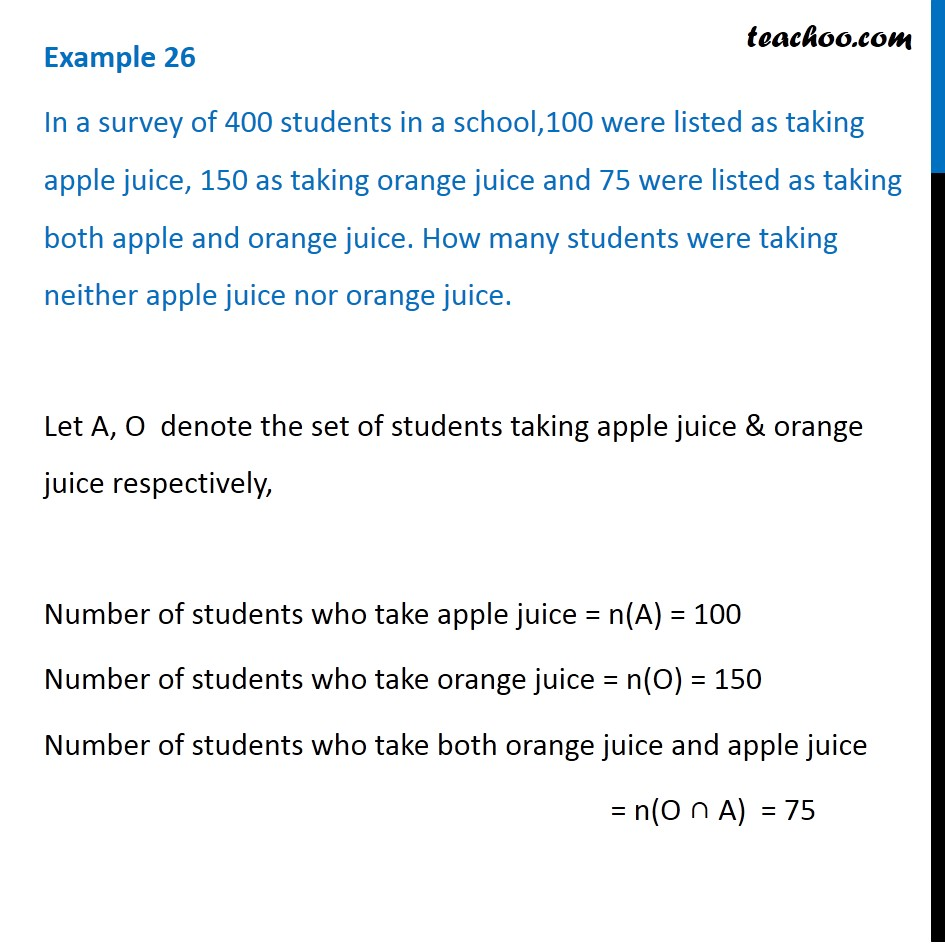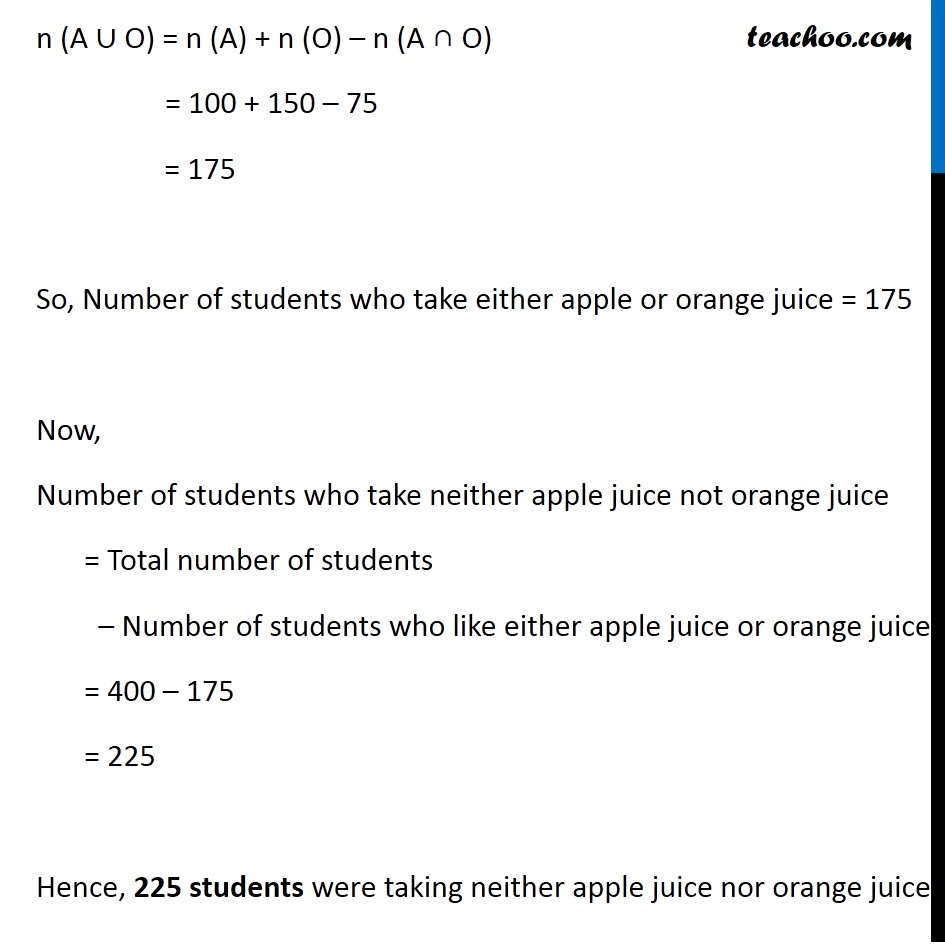1. Chapter 1 Class 11 Sets
2. Concept wise
3. Number of elements in set - 2 sets - (Using properties)

Transcript

Example 26 In a survey of 400 students in a school,100 were listed as taking apple juice, 150 as taking orange juice and 75 were listed as taking both apple and orange juice. How many students were taking neither apple juice nor orange juice. Let A, O denote the set of students taking apple juice & orange juice respectively, Number of students who take apple juice = n(A) = 100 Number of students who take orange juice = n(O) = 150 Number of students who take both orange juice and apple juice = n(O ∩ A) = 75 n (A ∪ O) = n (A) + n (O) – n (A ∩ O) = 100 + 150 – 75 = 175 So, Number of students who take either apple or orange juice = 175 Now, Number of students who take neither apple juice not orange juice = Total number of students – Number of students who like either apple juice or orange juice = 400 – 175 = 225 Hence, 225 students were taking neither apple juice nor orange juice.

Number of elements in set - 2 sets - (Using properties)